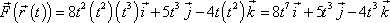# Vector field line integral

Fine the word done in moving a particle in the force field F=<2sin(x)cos(x), 0, 2z> along the path r=<t,t,t2>, 0≤t≤π

To do the line integral, I need to find F(r(t)), but I don't understand how to express it. For example I looked at the online notes provided here: http://tutorial.math.lamar.edu/Classes/CalcIII/LineIntegralsVectorFields.aspx

I don't understand howwas derived. I know how to do the rest.

SteamKing
Staff Emeritus
Homework Helper
Fine the word done in moving a particle in the force field F=<2sin(x)cos(x), 0, 2z> along the path r=<t,t,t2>, 0≤t≤π

To do the line integral, I need to find F(r(t)), but I don't understand how to express it. For example I looked at the online notes provided here: http://tutorial.math.lamar.edu/Classes/CalcIII/LineIntegralsVectorFields.aspx

I don't understand howwas derived. I know how to do the rest.
In the example, for the vector function F(x,y,z), you want to find the values of F(x,y,z) along the path r (x,y,z), which is expressed parametrically as:
r(x,y,z) = r(t) = (t, t2, t3)

Now F(x,y,z) = (8x2yz, 5z, -4xy), so everywhere you see an x, make the substitution x = t; everywhere you see y, y = t2; ditto z, z = t3

Thus F(x,y,z) = F(r(t)) = [8*t2*t2*t3, 5*t3, -4*t*t2] = [8t7, 5t3, -4t3]